### MEMO 2007 pojedinačno problem 3

Kvaliteta:
Avg: 3,0
Težina:
Avg: 6,0
Let$k$ be a circle and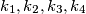$k_{1},k_{2},k_{3},k_{4}$ four smaller circles with their centres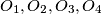$O_{1},O_{2},O_{3},O_{4}$ respectively, on$k$. For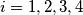$i = 1,2,3,4$ and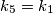$k_5=k_1$ the circles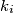$k_i$ and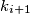$k_{i+1}$ meet at$A_i$ and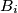$B_i$ such that$A_i$ lies on$k$. The points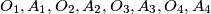$O_{1},A_{1},O_{2},A_{2},O_{3},A_{3},O_{4},A_{4}$ lie in that order on$k$ and are pairwise different.

Prove that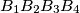$B_{1}B_{2}B_{3}B_{4}$ is a rectangle.
Izvor: Srednjoeuropska matematička olimpijada 2007, pojedinačno natjecanje, problem 3